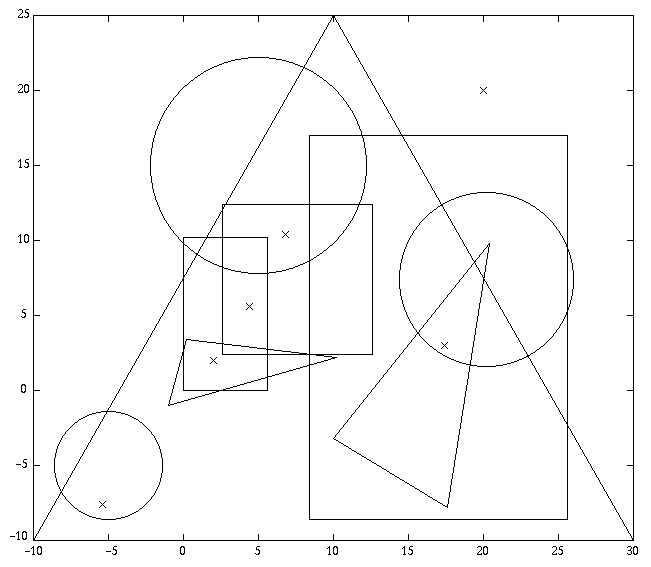# Programming contests

## ECN selejtező programozó csapatverseny, 2014. április 27.

April 27, 2014 10:30 AM – April 27, 2014 3:30 PM

# Points in Figures: Rectangles, Circles, and Triangles

Given a list of figures (rectangles, circles, and triangles) and a list of points in the x-y plane, determine for each point which figures (if any) contain the point.

## Input Specification

There will be n (≤ 10) figure descriptions, one per line. The first character will designate the type of figure (“`r`”, “`c`”, “`t`” for rectangle, circle, or triangle, respectively). This character will be followed by values which describe that figure:

• For a rectangle, there will be four real values designating the x-y coordinates of the upper left and lower right corners.
• For a circle, there will be three real values, designating the x-y coordinates of the center and the radius.
• For a triangle, there will be six real values designating the x-y coordinates of the vertices.

The end of the list will be signalled by a line containing an asterisk in column one.

The remaining lines will contain the x-y coordinates, one per line, of the points to be tested. The end of this list will be indicated by a point with coordinates 9999.9 9999.9; these values should not be included in the output.

Points coinciding with a figure border are not considered inside.

## Output Specification

For each point to be tested, write a message of the form

``` Point i is contained in figure j ```

for each figure that contains that point. If the point is not contained in any figure, write a message of the form

``` Point i is not contained in any figure ```

Points and figures should be numbered in the order in which they appear in the input.

## Sample Input

1. `r 8.5 17.0 25.5 -8.5`
2. `c 20.2 7.3 5.8`
3. `t -1.0 -1.0 10.1 2.2 .4 1.4`
4. `r 0.0 10.3 5.5 0.0`
5. `c -5.0 -5.0 3.7`
6. `t 20.3 9.8 10.0 -3.2 17.5 -7.7`
7. `r 2.5 12.5 12.5 2.5`
8. `c 5.0 15.0 7.2`
9. `t -10.0 -10.0 10.0 25.0 30.0 -10.0`
10. `*`
11. `2.0 2.0`
12. `4.7 5.3`
13. `6.9 11.2`
14. `20.0 20.0`
15. `17.6 3.2`
16. `-5.2 -7.8`
17. `9999.9 9999.9`
download as text file

## Output for Sample Input

1. `Point 1 is contained in figure 4`
2. `Point 1 is contained in figure 9`
3. `Point 2 is contained in figure 4`
4. `Point 2 is contained in figure 7`
5. `Point 2 is contained in figure 9`
6. `Point 3 is contained in figure 7`
7. `Point 3 is contained in figure 8`
8. `Point 3 is contained in figure 9`
9. `Point 4 is not contained in any figure`
10. `Point 5 is contained in figure 1`
11. `Point 5 is contained in figure 2`
12. `Point 5 is contained in figure 6`
13. `Point 5 is contained in figure 9`
14. `Point 6 is contained in figure 5`
15. `Point 6 is contained in figure 9`
download as text fileDiagram of sample input figures and points

University of Debrecen; Faculty of Informatics; v. 03/01/2019